3.2.11.2 Hydrodynamic Impact Ionization ModelNext: 3.2.11.3 Simplified Hydrodynamic Model Up: 3.2.11 Impact Ionization Previous: 3.2.11.1 Impact Ionization for

### 3.2.11.2 Hydrodynamic Impact Ionization Model

Having discussed some principal properties of bulk material within the DD approach impact ionization modeling of submicron FETs is addressed in this section.

The model by Quade et al.  used here is based on the assumption of a heated Maxwellian energetic distribution of the carriers. As impact ionization is a generically a hydrodynamic effect based on the carrier temperature the generation rates for direct semiconductors written as: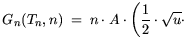exp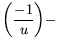erfc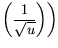(3.73)

and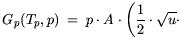experfc(3.74)

For indirect semiconductors, however, the formula reads: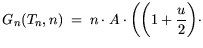erfc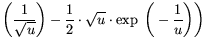(3.75)

and: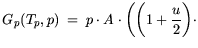erfc(3.76)

using: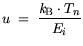(3.77)

The two sets of equations (3.76)(3.77) and (3.78)(3.79) are derived as two limiting cases from the same basic model as explained in . The difference arises from the allowed energies after the impact ionization process in the case of a direct and indirect semiconductor. erfc() is the complementary error function 1-erf(). Special care has to be taken for the implementation of the direct model to avoid numerical uncertainties, that may arise due to often encountered poor implementations of the complementary error function .

The lattice temperature dependence is modeled in the prefactor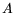using four parameters. This allows to include the two different temperature represented by GaAs and In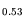Ga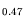As.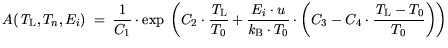(3.78)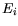is the threshold energy at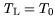= 300 K modeled as given above. For indirect semiconductors, the energyis the approximately the band gap, while for direct semiconductors, there is the difference, as shown in Table 3.31. Aspects of the parameter extraction are described in Section 3.6. Basically, the impact ionization generation rates are found by one-dimensional MC simulation as a function of the corresponding carrier temperatures using the potential profile obtained from a HEMT channel at high bias. The model parameters in (3.81) are then fitted to the generation rates obtained by the MC model as a function of carrier temperature. The model parameters thus contain all the assumptions made by the HD impact ionization model, which suggests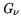to be a single valued function of the average carrier temperature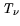. The values found useful can be found in Table 3.31.

Table 3.31: Modeled HD impact ionization rates for electrons and holes in III-V semiconductors.
 Material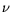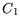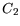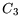E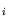[1/s] [eV] GaAs n 1.3e8 3.8 0.35 0.1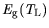+ 0.52 p+ 0.72 Al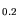Ga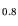As n 1e8 3.8 0.35 0.1+ 0.51 p+ 0.64 InGaAs n 2.3e8 3.8 0.35 0.1+ 0.41 p+ 0.6 InGaAs n 2e8 3.8 0.35 -0.1+ 0.3 p+ 0.51 In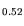Al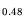As n 2e8 3.8 0.35 0.1+ 0.71 p+ 1.31 Si n 1.0493e8 3.823 0.34633 0.0922pThe data for the lattice-temperature dependence are obtained by comparison with the approach in the previous section, which suggests to model the threshold energy. For InGaAs the lattice temperature dependence is changed by the sign of the parameter, which leads to the increase of the impact ionization with lattice temperature.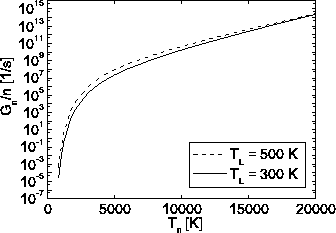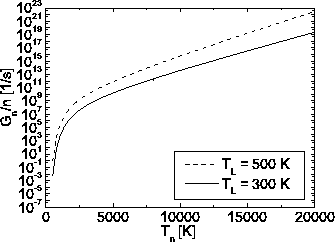Fig. 3.20 and Fig. 3.21 show the principal difference of impact ionization for GaAs representative for AlGaAs and InAlAs for all material compositions, and for InGaAs, while InGaAs shows a different behaviour (Fig. 3.21). It can be seen that for GaAs with increasing lattice temperaturesthe slope ofdrops. This leads to a crossing of the curves for carrier temperatures about= 20000 K, i.e., for average carrier energy of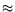2 eV. As will be shown in Fig. 3.30, some carriers reach energies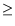1 eV already for relatively low bias of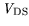= 2 V. For InGaAs, an opposite behavior is found: The generation increases as a function of, which causes hot electron reliability problems. If material compositions other than given in Table 3.31 are needed they can be interpolated between the materials.Next: 3.2.11.3 Simplified Hydrodynamic Model Up: 3.2.11 Impact Ionization Previous: 3.2.11.1 Impact Ionization for
Quay
2001-12-21